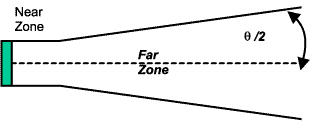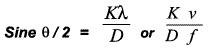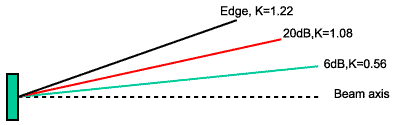Subscribe to our newsletter to receive the latest news and events from TWI:

# The beam spread of an ultrasonic probe in the far zone

In the far zone (also called the fraunhöfer) sound pulses spread out as they move away from the crystal.The angle of beam spread can be found by using this formula:where

 K = constant for the edge of the beam spread D = diameter of crystal λ = wavelength v = velocity of sound in material f = crystal frequency

From the formula it can be seen that:

• The larger the diameter, the smaller the beam spread
• The higher the frequency, the smaller the beam spread

The K value selected can actually vary according to the point at which the beam spread is calculated. The different values to be used are shown below.

At the edge of the beam, the sound energy is 1/10 (-20dB) of the energy at the beam centre, and K is 1.08. If the extreme edge of the sound beam is used to work out beam spread angles, then K is 1.22.